# Understanding the Meaning of Random

One of the most overlooked concepts of Hearthstone is the concept of Randomness. By understanding Chance, we can make the best decisions.

## Introduction

One of the most overlooked concepts of Hearthstone is the concept of Randomness, probably because most players are reading the portion of the text after the “random” which is “Deal X damage.”

So in their minds, playing this card is always a good thing, right?  Not always.  Before we dive any further into Hearthstone specifics, let’s do a quick review on what Randomness really is.

## Probability 101

Recall back to that math class where you learned about Probability. (Haven’t taken that class yet? Great, now you will be ahead of the game and can apply Hearthstone to your schoolwork.  Who said games can’t be educational?)

If I have 2 apples and 2 oranges in a bag, what is the probability that I randomly choose an apple?  The easiest way to do this is to list out all the possible outcomes and add the winners then divide by the total number of fruits.  We could:

Choose apple “A1”

Choose apple “A2”

Choose orange “O1”

Choose orange “O2”

To win, we need either A1 or A2, so that is 2.  Total number of outcomes is 4, so that is 2/4 = 50%. You can quickly see how tedious this could be for a more complex situation.  We can generalize this by simply calculating the total number of apples and dividing it by the total number of fruits in the bag, which is 2 apples / 2 + 2 fruits, so 2/4 = 50%.  There is a 50% chance I will pick an apple.

What if there were 3 apples and 2 oranges?  Then it will be 3 / (3 + 2) = 3 / 5 = 60%.

## Probability 102

Let’s turn it up a notch.  What if I had an apple, an orange, a banana, and a watermelon in a bag, and I had to randomly pick 2 fruits from the bag, what are the chances I will pick an apple and an orange? If you’re thinking “50% because you’re choosing 2 fruits out of 4 fruits” you’re mistaken. To correctly analyze what the probability is, we must go through every possible scenario and add up our “winners.”

Scenario 1: We pick the apple first, then we pick the orange.  What are the chances of us picking the apple? ¼. What about the orange? Well, since we already picked the apple, there are only 3 fruits left in the bag, so ?.  We multiply these together to get 1/12.

Scenario 2: We pick the orange first, then the apple.  What are the chances of us picking up the orange? ¼. What about the apple? Sounds familiar? It is the same scenario as above, which leads us to 1/12. Do we have any other winning situations? No we don’t, so we can add our winners up: 1/12 + 1/12 = 2/12 = ? = 16.67%.

## Probability 103

Suppose you’re curious what happens if we’re in the same scenario as Probability 102, except after the first time we picked a fruit from the bag, we put it back.  What’s the probability then?  Same concept:  we walk through our winning scenarios and add them.

Scenario 1: We pick an apple, put it back, then pick an orange.  The first event is ¼, but then we put it back, so the second event is also ¼. Multiply these together and we get 1/16.

Scenario 2: We pick an orange, put it back, then pick an apple.  Same thing: First event is ¼, put the orange back, and pick the apple which is ¼. Multiply together and we get 1/16.

Is there any other possible scenarios? No, so we add these together: 1/16 + 1/16 = 2/16 = 12.5%.  That’s different from what we learned in Probability 102, where it was 16.67%! This difference is known as “With replacement” vs “Without replacement.”  In 102 we didn’t replace the fruit we picked the first time (without replacement), and in 103 we replaced it (with replacement).  This will change the outcome of probabilities, depending on the circumstances.DreadLo

Great, now we understand the concepts.  From here on I will explain some situations and apply what we have learned.

## Scenario 1: Tinkmaster Overspark

Suppose you are in this hypothetical situation:

Your opponent has a 8/8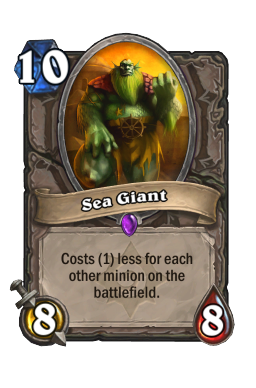sea-giant, a 2/3 minion and a 2/1 minion, and you have a 3/3 minion.  You have a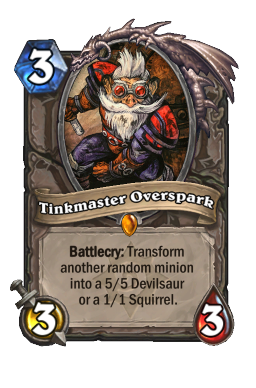tinkmaster-overspark in your hand.  What is the probability you will transform one of the opponent’s minion?

Like in Probability 101, we have 3 apples (opponent’s minions) and 1 orange (your minion).  3 / (3 + 1) = 75%.  There is a good chance we will transform one of their minions.

What about the probability that we transform the Sea Giant?  In this case, we have 1 apple (the sea giant) and 3 oranges (Not the sea giant), so 1 / (1 + 3) is 25%.

But wait that’s not all that Tinkmaster does.  It could either be a 5/5 devilsaur or a 1/1 squirrel.  So is this a good idea? Let’s walk through all the scenarios.

We already calculated the probability that transforming the Sea Giant will be 25%.  From my understanding (since I don’t work at Blizzard and don’t have any idea what the exact probability is for changing into a 5/5 vs a 1/1) I will assume it is a 50/50 split.  Multiply 25% by 50% and you get 12.5% chance the Sea giant will be a 5/5, and 12.5% it will be a 1/1.  For the sake of simplicity, I would argue that both of these are a Win scenario.

How about that 2/3 minion? By now you should have noticed a pattern that the probability of transforming this particular minion is the same as transforming the Sea Giant, which is 25%.  The difference here is whether or not we like it being a 5/5 or a 1/1.  The probability is still the same for both: 12.5%.  However I would like to think a 5/5 is a LOSE situation, and 1/1 is a WIN situation.

And the 2/1 minion? Same probabilities: 12.5% chance it’s a LOSE situation where it turns into a 5/5, and 12.5% chance it’s a WIN situation with a 1/1 transformation.

How about your 3/3 minion? Same probabilities: 12.5% it’s a WIN situation with a 5/5, and 12.5% with a LOSE situation with a 1/1.

Let’s go back to our original question.  What is the probability we would transform one of the opponent’s minions?  Think about this again.  Is this the right question we want to ask, or should we ask a much more specific question, say, “What is the probability of a WIN situation?”  If we add all of them up, 12.5% (Sea Giant turns to 5/5) + 12.5% (Sea Giant turns to 1/1) + 12.5% (their 2/3 minion turns to 1/1) + 12.5% (their 2/1 minion turns into 1/1) + 12.5% (your 3/3 becomes a 5/5) = 62.5%.  That is a pretty good chance you are in a WIN scenario.

But suppose that’s not the question you want to ask because that includes chances that the Sea Giant is still 8/8.  He is our biggest threat, and changing a 2/3 to a 1/1 really isn’t a WIN situation, nor is changing our 3/3 to a 5/5.  We really just don’t want that 8/8.  That means our only WIN situation is transforming the Sea Giant to anything.  We’ve already calculated that to be 25%.  Is 25% something you are willing to bet on?

Or we can further say “Transforming a 8/8 to a 5/5 may still hurt me in the end.  I want it to be a 1/1!” We’ve calculated that as well, which is 12.5%.  Ouch!

So let’s ask ourselves, what now?  What if we attacked the 2/3 with our 3/3 first? Now we have ourselves a whole new situation: Opponent has 8/8, 2/1 You have 3/1 Without going into all the math, we now have a ? (16.67%) chance that the Sea Giant will transform into a 1/1.  That’s better than 12.5%! Let’s further pretend you have Mana Coil and you kill off the 2/1.  Now there is only the Sea Giant and your 3/1 remaining on the board.  Now we have a ¼ (25%) chance it will get transformed to a 1/1.  I’m liking that a lot better!

Let’s recap what we’ve learned:

2. Randomness really depends on what is currently in play the odds can totally change later.
3. Because of #2, order of operation matters.  You might want to change the board slightly to increase the odds to your favor.  One way to do this is to decrease the number of minions the opponent has.

## Scenario 2: Brawl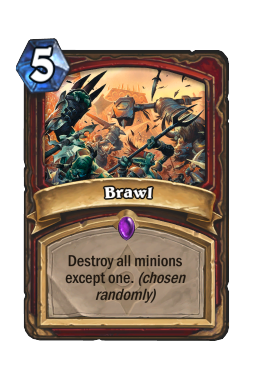brawl destroys all minions on the board except one.  Let’s play this hypothetical situation out.  For all practical purposes, let’s assume your opponent has 4 minions on the board and they are all 3/3.  You have no minions; nada.  You have a Brawl in your hand.  What should you do?  Obviously playing the Brawl will eliminate all but one 3/3 minion on the board.

Remember what we’ve learned from Scenario 1.  What if you could change the situation? What if you could also play a minion first?

Before we go into our different scenarios, let’s remember one vital concept:  with randomness, we are concerned with the OUTCOME.  Let’s suppose your minion was a 3/1 Wolfrider.  We can simply play the Brawl and the outcome is a 3/3 enemy minion, and we can play the wolfrider to eliminate the enemy minion, resulting in a board wipe.  Maybe this is what we want, maybe not.

What if we want our outcome to be a situation where not only does the opponent have no minions, but we also have a minion standing?  There’s only one way to do that with Brawl, and that’s to put in your minions.

So now you played your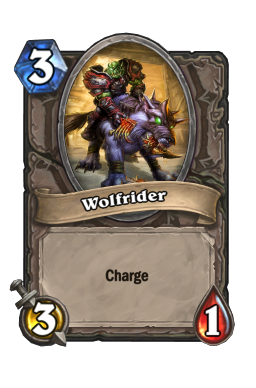wolfrider and you play brawl.  The chances of your Wolfrider surviving is 1 / (4 + 1) = 20%.  That’s not necessarily good.

What if instead you had a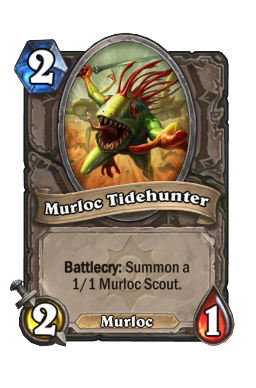murloc-tidehunter?  It summons another 1/1 minion, and now your chances are 2 / (4 + 2) = 2 / 6 = 33.33%.  It’s better than 20%!  Similarly if you played two Murloc Tidehunters, you now have a 4 / (4 + 4) = 4 / 8 = 50% chance that your opponent has no minions while you have one standing!

Let’s recap this scenario:

1. In Scenario 1, I mentioned you can always change the odds to your favor by decreasing the number of enemy minions.  Likewise, you can change the odds by adding more of your minions.

## Scenario 3: Multi-shot

Here’s the situation: Your opponent has a 2/3, 4/3 and 2/1.  You have 7 mana crystals with the following hand: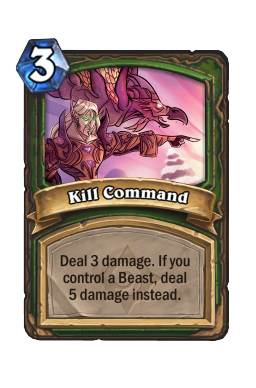kill-command,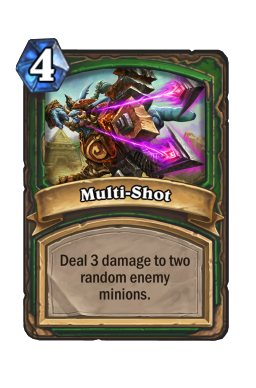multi-shot, and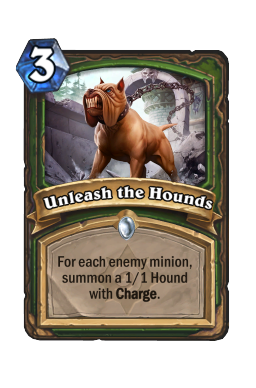unleash-the-hounds.  What is your move?

I would argue the following:

1. Unleash the Hounds.
2. Use one to kill the 2/1
3. Multi-Shot
4. You now have 2 1/1 Hounds which have not yet attacked. Hit the hero.

Let’s talk about Split Shot:  It chooses two targets WITHOUT replacement (see Probability 103) and deals 3 damage.  The most optimal use of Split Shot in this case would be to take out the 2/3 and the 4/3.  So let’s go through the scenario where we play Split Shot first:  (Chance of picking the 2/3) * (Chance of picking the 4/3) + (Chance of picking the 4/3) * (Chance of picking the 2/3) = (? * ½) + (? * ½) = 33.33%.  That means the other 66.67% of the time, we may accidentally kill the 2/1 instead.

By using Unleash the Hounds, we can eliminate that possibility, so there are only two targets left (the 2/3 and 4/3) and we know the possibility of hitting both of these is 100%.  Simply removing the 2/1 threat can guarantee we clear the board and get our maximum reward.

## Scenario 4: Arcane Missiles

Was that an urge to learn about situations WITH replacement I sense? No problem, Arcane Missiles is your scenario.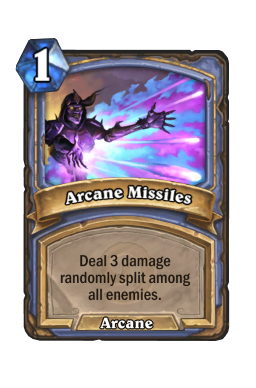arcane-missiles fires 3 missiles that does 1 damage each.  So suppose you have yourself this scenario:  Your opponent has a 3/3.  What are the chances you kill the 3/3?

Each Arcane Missile has a ½ chance of hitting the 3/3 (remember, the hero is also a valid target).  So the probability would be ½ * ½ * ½ = 12.5%.  Arcane missile itself does a lousy job getting rid of a 3/3.

Suppose you can also the mana to throw out fireblast.  Now the minion is a 3/2.  What’s the probability now?  It might get confusing because now it has 2 health.  Not to worry, you just need to ask the correct question:  What is the probability I will hit the Hero once and the minion twice (mainly, the minion twice)?

You may be tempted to list all the scenarios out because it seems easier.  M denotes hitting the minion, H denotes hitting the hero:

M, M, H

M, H, M

M, H, H

H, M, M

H, M H

H, H, M

H, H, H

Thus there are 3 winners of the 7 scenarios with the scenarios of two M hits, so the probability is 3/7 = 42.85%.  However this is incorrect: you are forgetting the scenario:

M, M, M

But the minion only has 2 life! How is that possible?  That is because the behavior of the missile that goes and hits the hero doesn’t change probability; the last damage just gets redirected to the hero by the game.  You can think of it as “It should have hit the minion, but since it is dead, it will go and hit the Hero.”  That means there is an extra (M, M, H) scenario, thus 4 / 8 = 50%.  However it is easier if you write (M, M, M) and count it on its own instead of writing (M, M, H) twice.

Alternatively we can think of it mathematically.  The math says that after the first two missiles hit the minion, we don’t care what the third outcome is, but it could have been the minion if it were there.  This math is shown by:

(Chance of hitting minion) * (Chance of hitting minion, thus it’s dead) * (100% because we don’t care! it’s dead!) => (½ * ½ * 1) = 25%.  Add this your other scenarios: M, H, M and H, M, M = (Chance of hitting minion) * (Chance of missing) * (Chance of hitting Minion) + (Chance of missing) * (Chance of hitting the minion) * (Chance of hitting the minion) = (½ * ½ * ½) + (½ * ½ * ½) = 25%.  The result is 50%; there is actually a 50% of killing the 3/2 minion.  This is significantly better than if you played Arcane Missiles by itself.

Let’s recap this confusing topic:

1. Order matters.  Because the minion could be dead in the middle of the arcane missile spell, the math has to incorporate that.
2. To do this, simply write out your scenarios as (What you want, what you want, what you want), multiply those probabilities together, then add the winners.  In our case we wanted (M, M, Anything!), (M, H, M) and (H, M, M)

## Scenario 4.1: Arcane Missile with multiple targets that can die right away

Let’s try a harder scenario: You are against a hero, a 2/1 and a 2/2 and you really want to kill that 2/2.  What’s the probability?

Do we want to list out the scenario? H is hero, M1 is Minion with 2/1 life, and M2 is Minion with 2/2 life:

H, H, H

H, H, M1

H, H, M2

H, M1, M2…

This is starting to get pretty tedious and that 90 second ticker isn’t going to wait for you, and we learned in Scenario 4, that it could be misleading! Help!

Not to worry: let’s do the math! EDIT: My math was wrong! Thanks to ziAppi for catching this error!

We have 5 scenarios:

a) Killing 2/2 straight (M2, M2, Anything!)

1/3 * 1/3 * 1 = 11.11%

b) Killing 2/1 first and then 2/2 (M1, M2, M2)

1/3 * 1/2 (because the 2/1 is dead now) * 1/2 = 8.33%

c) Hitting 2/2, then 2/1, then 2/2 (M2, M1, M2)

1/3 * 1/3 * 1/2 (because the 2/1 is dead now) = 5.56%

d) Hitting 2/2, then hero, then 2/2 (M2, H, M2)

1/3 * 1/3 * 1/3 = 3.70%

e) Hitting hero, then 2/2, then 2/2 (H, M2, M2)

1/3 * 1/3 * 1/3 = 3.70%

Adding them together you get 32.4%

What about if you just want to kill the 2/1? Chance of (M1, Anything! Anything!) + Chance of (Miss, M1, Anything!) + Chance of (Miss, Miss, M1) = (? * 1 * 1) + (? * ? * 1) + (? * ? * ?) = ? + 2/9 + 4/27 = 70.37%

What about both killing the 2/2 and 2/1, or essentially, not hitting the Hero at all? Chance of (M1, M2, M2) + (M2, M1, M2) + (M2, M2, M1) = (? * ½ (because the 2/1 is dead, we only have two targets!) * ½) + (? * ? * ½) +  (? * ? * ½ (because the 2/2 is dead, we only have two targets!)) = 19.44%.

Congratulations, that was a scenario with replacement AND without replacement!

## Conclusion

Hopefully by now you are either much more enriched with this knowledge or you are even more confused and don’t ever want to mess with randomness.  If the latter is true, I don’t blame you, but I would suggest you not incorporate any random cards into your deck.  As you can see, random card effects have drastic changes in outcome depending on the situation, and thus are very situational cards.  I would highly not recommend building a deck around random cards.

For those who have a better understanding, I hope now you can find better use in your cards and know how to increase your odds to get out of a pinch.  Recall that order matters, increasing your own odds and decreasing your opponent’s odds could potentially turn the tables around.  But remember, at the end of the day, it’s all up to Blizzard’s Random Number Generator.

Let me know if you liked this guide or not.  Please feel free to leave comments with feedback, or if you would like a guide with more scenarios or combos that would help your game.